Tamilnadu State Board New Syllabus Samacheer Kalvi 11th Maths Guide Pdf Chapter 12 Introduction to Probability Theory Ex 12.3 Text Book Back Questions and Answers, Notes.

## Tamilnadu Samacheer Kalvi 11th Maths Solutions Chapter 12 Introduction to Probability Theory Ex 12.3

Question 1.
Can two events be mutually exclusive and independent simultaneously?
When A and B are independent
P(A ∩ B) = P(A) P(B)
But when A and B are mutually
Exclusive P(A ∩ B) = 0Question 2.
If A and B are two events such that
P ( A ∪ B ) = 0.7 , P (A ∩ B) = 0.2 , and P (B) = 0.5 , then show that A and B are independent.
Given A and B are twp events such that
P(A ∪ B) = 0.7, P(A ∩ B) = 0.2 and P(B) = 0.5
To prove A and B are independent it is enough to prove
P(A ∩ B) = P(A) . P(B)
P(A ∪ B) = P(A) + P(B) – P(A ∩ B)
0.7 = P (A) + 0.5 – 0.2
0.7 = P(A) + 0.3
P(A) = 0.7 – 0.3 = 0.4
P(A) . P(B) = 0.4 × 0.5 = 0.20
= P(A ∩ B)
∴ P(A∩B) = P(A) . P(B)
∴ A and B are independent.

Question 3.
If A and B are two independent events such that P(A ∪ B) = 0.6, P(A) = 0.2, find p(B).
Given A and B are independent.
⇒ P(A ∪ B) = P(A).P(B)
Here P(A ∪ B) = 0.6 and P(A) = 0.2
To find P(B):
Now, P(A ∪ B) = P(A) + P(B) – P(A ∩ B)
(i.e.,) P(A ∪ B) = P(A) + P(B) – P(A) . P(B)
(i.e.,) 0.6 = 0.2 + P(B) (1 – 0.2)
P(B) (0.8) = 0.4
⇒ P(B) = $$\frac{0.4}{0.8}=\frac{4}{8}=\frac{1}{2}$$ = 0.5Question 4.
If P(A) = 0.5, P(B) = 0.8 and P(B/A) = 0.8, find P(A/B) and P(A ∪ B).
Given P(A) = 0.5, P(B) = 0.8
and P(B/A) = 0.8P(A ∩ B) = P(B/A) P(A)
Substituting in equation (1) we getP(A/B) = 0.5
P(A ∪ B) = P(A) + P(B) – P(A ∩ B) ………. (2)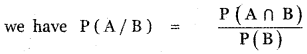P(A ∩ B) = P(A/B) . P(B)
= 0.5 × 0.8
P(A ∩ B) = 0.40
(2) ⇒ P(A ∪ B) = 0.5 + 0.8 – 0.40
= 1.3 – 0.40
P(A ∪ B) = 0.90Question 5.
If for two events A and B, P(A) = $$\frac{3}{4}$$ P(B) = $$\frac{2}{5}$$ and A ∪ B = S (sample space), find the conditional probability p (A/B).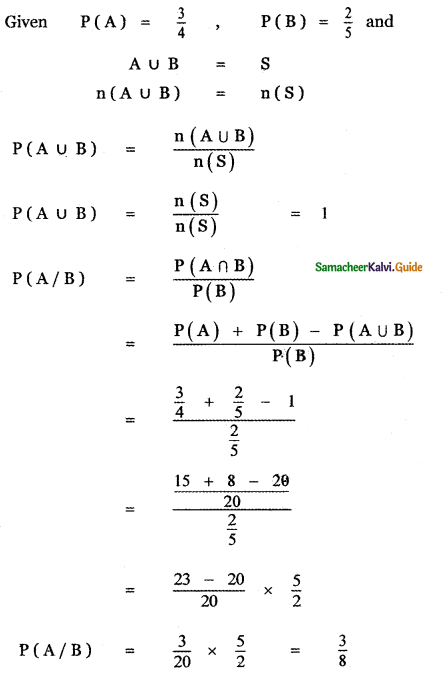Question 6.
A problem in Mathematics is given to three students whose chances of solving it are $$\frac{1}{3}$$, $$\frac{1}{4}$$ and $$\frac{1}{5}$$.
(i) What is the probability that the problem is solved?
(i) What is the probability that the problem is solved?
Let A1 denote the event of that first student solves the problem.
A2 denote the event that second student solves the problem.
A3 denote the event that third student solves the problem.
Given P(A1) = $$\frac{1}{3}$$, P(A2) = $$\frac{1}{4}$$ P(A3) = $$\frac{1}{5}$$
We note that A1, A2, A3 are independent events.
The problem will be solved if atleast one of them
solves it we have to find P(A1 ∪ A2 ∪ A3)
Probability of at least one solves the problem = 1 – Probability of no one solving it
P(A1 ∪ A2 ∪ A3) = 1 – P(A̅1 ∪ A̅2 ∪ A̅3)
= 1 – P(A̅1) . P(A2) . P(A3)
A1, A2, A3 are independent then A̅1, A̅2, A̅3 are also independent.
= 1 – [1 – p(A1)] [1 – P(A2)] [1 – P(A3)]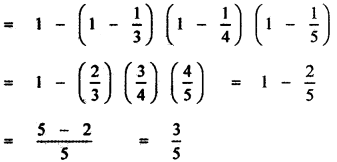(ii) What is the probability that exactly one of them will solve it?
p(A̅1) = 1 – P(A1) = 1 – $$\frac{1}{3}$$ = $$\frac{2}{3}$$
p(A̅1) = 1 – P(A2) = 1 – $$\frac{1}{4}$$ = $$\frac{3}{4}$$
p(A̅1) = 1 – P(A3) = 1 – $$\frac{1}{5}$$ = $$\frac{4}{5}$$
Probability of Exactly one student solving the problem[Probability of exactly one student solving the problem = Probability [(A1 solving the problem and A2, A3 non solving the problem) or (A1, A3 non solving and A2 solving) or (A1, A2 solving and A3 non solving)]Question 7.
The probability that a car being filled with petrol will also need an oil change is 0.30; the probability that it needs a new oil filter is 0.40; and the probability that the both the oil and filter need changing is 0.15.
(i) If the oil had to be changed, what is the probability that a new oil filter is needed?
(ii) If a new oil filter is needed, what is the probability that the oil has to be changed?
Let A be the event of changing oil, B be the event of changing oil filter.
Given P(A) = 0.30, P(B) = 0.40, P(A ∩ B) = 0.15

(i) Probability of new oil filter B needed when the oil A changed is(ii) Probability of oil A changed when new oil filter B is changed is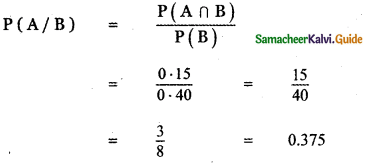Question 8.
One bag contains 5 white and 3 black balls. Another bag contains 4 white and 6 black balls. If one ball is drawn from each bag, find the probability that
(i) both are white
(ii) both are black
(iii) one white and one black
First Bag contains 5 white and 3 black balls Total number of balls in the first bag 8 Second Bag contains 4 white and 6 black halls Total number of balls in the second bag = 10
One ball is drawn from each bag.

(i) Probability of both are white:
P (getting both are white) = P (getting white ball from the first bag) × P (getting the white ball from the second bag)(ii) Probability of both are black:
P (getting both are black) = P (getting black ball from the first bag) × P (getting the ball from the second bag)(iii) Probability of one white and one black.
P (getting one white and one black) = P ( getting one white from the first bag or one white from the second bag) + P (getting one black from the first bag or one black from the second bag)Question 9.
Two-thirds of students in a class are boys and the rest girls. It is known that the probability of a girl getting the first grade is 0.85 and that of boys is 0.70. Find the probability that a student chosen at random will get first-grade marks.
Let G be the event of choosing a boy and G be the event of choosing a Girl.
Given P(B) = $$\frac{2}{3}$$, P(G) = $$\frac{1}{3}$$
Let B1 be the event of a boy getting first grade
P(B1) = 0.70
Let G1 be the event of a girl getting first grade
P(G1) = 0.85
Probability of a. student getting a first grade = Probability of a boy getting first grade or Probability
of a Girl getting first grade
= P(B) × P(B1) + P(G) × P(G1)
= $$\frac{2}{3}$$ × 0.70 + $$\frac{1}{3}$$ × 0.85
= $$\frac{1.4+0.85}{3}$$
= $$\frac{2.25}{3}$$ = 0.75

Question 10.
Given P(A) = 0.4 and P(A ∪ B) = 0.7 Find P(B) if
(i) A and B are mutually exclusive
(ii) A and B are independent events
(iii) P(A/B) = 0.4
(iv) P(B/A) = 0.5
P(A) = 0.4, P(A ∪ B) = 0.7
(i) When A and B are mutually exclusive
P(A ∪ B) = P(A) P(B)
(i.e.,) 0.7 = 0.4 + P(B)
0.7 – 0.4 = P(B)
(i.e.,) P(B) = 0.3

(ii) Given A and B are independent
⇒ P(A ∩ B) = P(A). P(B)
Now, P(A ∪ B) = P(A) + P(B) – P (A ∩ B)
(i.e.,) 0.7 = 0.4 + P(B) – (0.4) (P(B))
(i.e.,) 0.7 – 0.4 = P(B) (1 – 0.4)
0.3 = P (B) 0.6
⇒ P(B) = $$\frac{0 \cdot 3}{0 \cdot 6}=\frac{3}{6}$$ = 0.5

(iii) P(A/B) = 0.4
(i.e.,) $$\frac{P(A \cap B)}{P(B)}$$ = 0.4
⇒ P(A ∩ B) = 0.4 [P(B)] …………. (i)
But We know P(A ∪ B) = P(A) + P(B) – P(A ∩ B)
P(A ∩ B) = P(A) + P(B) – P(A ∪ B)
⇒ P(A ∩ B) = 0.4 + P(B) – 0.7
= P(B) – 0.3 …………. (ii)
from (i) and (ii) (equating R.H.S) We get
0.4 [P(B)] = P(B) – 0.3
0.3 = P(B) (1 – 0.4)
0.6 (P(B)) = 0.3 ⇒ P(B) = $$\frac{0.3}{06}=\frac{3}{6}$$ = 0.5

(iv) P(B/A) = 0.5
(i.e.,) $$\frac{P(A \cap B)}{P(A)}$$ = 0.5
(i.e.,) P(A ∩ B) = 0.5 × P(A)
= 0.5 × 0.4 = 0.2
Now P(A ∪ B) = P(A) + P(B) – P(A ∩ B)
⇒ 0.7 = 0.4 + P(B) – 0.2
⇒ 0.7 = P(B) + 0.2
⇒ P(B) = 0.7 – 0.2 = 0.5Question 11.
A year is selected at random. What is the probability that
(i) it contains 53 Sundays
(ii) it is a leap year which contains 53 Sundays.
Probability of year being a leap year = $$\frac{1}{4}$$
Probability of year being non – leap year = $$\frac{3}{4}$$

(i) It contains 53 Sundays:
A non – leap year has 365 days.
365 days = 52 weeks + 1 day.
52 weeks contain 52 Sundays.
In order to get 53 Sundays in a non – leap year the remaining I day must be a Sunday.
Remaining one day may be Sunday or Monday or Tuesday or Wednesday or Thursday or Friday or Saturday.
Probability of getting Sunday from the remaining one day = $$\frac{1}{7}$$
A leap year has 366 days.
366 days = 52 weeks + 2 odd days
52 weeks contain 52 Sundays.

In order to get 53 Sundays in a leap year the remaining 2 days must contain a Sunday. Remaining Two days may be

S = { (Sunday, Monday), (Monday, Tuesday), (Tuesday, Wednesday), (Wednesday, Thursday), (Thursday, Friday ), ( Friday, Saturday), (Saturday, Sunday) }
n(S) = 7

Let A be the event set of getting a Sunday then
A = { (Sunday, Monday), ( Saturday , Sunday) }
n(A) = 2

P (getting a Sunday from the remaining 2 days)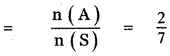P(getting 53 Sundays in a year) = P(getting a leap year) × P(getting a Sunday from the remaining 2 days) + P(getting a non-leap year) × P(getting a Sunday from the remaining 1 day)∴ Probability of getting 53 Sundays in a year = $$\frac{5}{28}$$

(ii) A leap year has 366 days
$$\frac{366}{7}$$ = 52 weeks + 2 days
In 52 weeks, we get 52 Sundays.
From the remaining two days we should get one Sunday, the remaining two days can be any one of the following combinations.
Saturday and Sunday, Sunday and Monday, Monday and Tuesday, Tuesday and Wednes¬day, Wednesday and Thursday, Thursday and Friday, Friday and Saturday of the seven combinations two have Sundays.
∴ (Probability of getting a Sunday = $$\frac{2}{7}$$
Selecting a leap year = $$\frac{1}{4}$$
{∴ In every four consecutive years we get one leap year}
∴ Probability of getting 53 Sundays = $$\frac{2}{7} \times \frac{1}{4} \times \frac{1}{14}$$Question 12.
Suppose the chances of hitting a target by a person X is 3 times in 4 shots, by Y is 4 times in 5 shots, and by Z is 2 times in 3 shots. They fire simultaneously exactly one time. What is the probability that the target is damaged by exactly 2 hits?
Probability X hitting the targent P (X) = $$\frac{3}{4}$$
Probability Y hitting the targent P (Y) = $$\frac{4}{5}$$
Probability Z hitting the targent P (Z) = $$\frac{2}{3}$$
P(X̅) = 1 – P(X) = 1 – $$\frac{3}{4}$$ = $$\frac{1}{4}$$
P(Y̅) = 1 – P(Y) = 1 – $$\frac{4}{5}$$ = $$\frac{1}{5}$$
p(Z̅) = 1 – P(Z) = 1 – $$\frac{2}{3}$$ = $$\frac{1}{3}$$